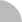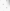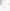臺灣博碩士論文加值系統

(23.20.20.52) 您好！臺灣時間：2022/01/24 18:28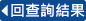:::

詳目顯示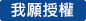: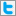Twitter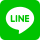•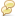被引用:0
•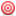點閱:139
•評分: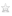•下載:0
•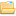書目收藏:0
 一個圖G的k圈填充是圖G邊中不互相相同的k圈集合。然而並不是所有的圖都可以被k圈填充，我們將剩下的邊集合所導出的子圖稱為這個裝填的殘留。能夠將殘留的部分邊數最少的裝填又稱為是最大的裝填。此時殘留的部分自然是最小殘留。 對應於裝填的觀念，我們也可考慮用k圈來覆蓋圖G。不夠的部分需加一些補丁， 如果補丁所用的邊數為最少，則此為最小覆蓋。 研究填充與覆蓋的問題已有百多年的歷史了，而最有名的題目就是完全圖的3圈填充。在此論文中我們著眼於5圈及 6圈的填充而考慮的圖形為平衡的完全多部分圖及兩個完全圖的卡笛森運算。
 A $k$-$cycle$ $packing$ of a graph $G$ is a set of edge-disjoint $k$-cycles in $G$. A $k$-cycle packing $C$ is $maximum$ if $|C|\geq |C''|$ for all other $k$-cycle packings $C''$ of $G$. The $leave$ $L$ of a packing $C$ is the subgraph induced by the set of edges of $G$ that does not occur in any $k$-cycle of the packing $C$. Therefore a maximum packing has a minimum leave. A $k$-$cycle$ $covering$ of $G$ is a set of edge-disjoint $k$-cycles $\mathcal{C}$ such that each edge of $E(G)$ occurs in at least one $k$-cycle in $\mathcal{C}$. A $k$-cycle covering $\mathcal{C}$ is $minimum$ if $|\mathcal{C}|\leq |\mathcal{C''}|$ for all other $k$-cycle coverings of $G$. The graph induced by the set of edges which are added to $G$ in the covering is called the $padding$ of the covering. Clearly, a minimum padding can be obtained in a minimum covering. The study of packing and covering problem commenced more than one hundred years ago. The most famous one is a $3$-cycle packing (respectively, covering) of the complete graph $K_{v}$. In case that the leave of a 3-cycle packing is an empty graph, we have a Steiner triple system of order $v$. It is indeed very closely related to the study of combinatorial designs. In this thesis, we shall focus on the study of 5-cycle and 6-cycle packings (respectively, coverings). By considering $G$ as the balanced complete multipartite graphs $K_{m(n)}$ and the Cartesian product of two complete graphs $K_{m}\times K_{n}$, we have obtained several results which will be presented in chatpers two to six.
 Abstract i Contents iii 1 Introduction 1 1.1 Motavation 1 1.2 The preliminaries in Graph Theory 3 1.3 The preliminaries in Combinatorial Design 5 1.4 Some results in cycle packing 8 2 Packing $K_{m(n)}$ with $5$-cycles 10 3 Packing $K_{m(n)}$ with $6$-cycles 17 4 Packing $K_{m}\times K_{n}$ with 5-cycles 28 5 Packing $K_{m}\times K_{n}$ with 6-cycles 36 6 Minimum covering 42 6.1 Covering $K_{m}\times K_{n}$ with 5-cycles 43 6.2 Covering $K_{m(n)}$ and $K_{m}\times K_{n}$ with 6-cycles 45 Conclusion 50 Bibliography 51
 \bibitem{chow1}\label{chow1}P. Adams, D. E. Bryant, and A. Khodkar, 3,5-cycle Decompositions,J. Combin. Des. 6 (1998), no. 2, 91--110.\bibitem{chow2}\label{chow2}B. Alspach and H. Gavlas, Cycle decompositions of $K\sb n$ and$K\sb n-I$, J. Combin. Theory Ser. B 81 (2001), no. 1, 77--99.\bibitem{chow3}\label{chow3}B. Alspach and S. Marshall, Even cycle decompositions of completegraphs minus a $1$-factor, J. Combin. Des. 2 (1994), no. 6,441--458.\bibitem{chow4}\label{chow4}B. Alspach and B. N. Varma, Decomposing complete graphs intocycles of length $2p\sp{e}$, Ann. Discrete Math. 9 (1980),155--162.\bibitem{chow5}\label{chow5}I. Anderson, Combinatorial Designs: Construction Methods, England,1990.\bibitem{chow6}\label{chow6}D. J. Ashe, C. A. Rodger, and H. L. Fu, All 2-regular leaves ofpartial 6-cycles system, in preprints.\bibitem{chow7}\label{chow7}E. Bell, Decomposition of a complete graph into cycles of lengthless than or equal to 50. M. Sc. Thesis, Auburn University, 1991.\bibitem{chow8}\label{chow8}J. C. Bermond, C. Huang, and D. Sotteau, Balanced cycle andcircuit designs: Even cases, Ars Combin. 5(1978), 293--318.\bibitem{chow9}\label{chow9}J. C. Bermond, and D. Sotteau, Balanced cycle and circuit designs:Odd cases. Proc. Colloq. Oberhof Illmenau, (1978), 11--32.\bibitem{chow10}\label{chow10}E. J. Billington, H-L. Fu and C. A. Rodger, Packing completemultipartite graphs with 4-cycles, J. Combin. Des. {\bf 9} (2001),107--127.\bibitem{chow11}\label{chow11}E. J. Billington, H-L. Fu and C. A. Rodger, Packing $\lambda$-foldcomplete multipartite graphs with 4-cycles, in preprints.\bibitem{chow12}\label{chow12}D. Bryant and A. Khodkar, Maximum packings of $K\sb v-K\sb u$ withtriples, Ars Combin. {\bf 55} (2000), 259--270.\bibitem{chow13}\label{chow13}N. J. Cavenagh and E. J. Billington, Decompositions of completemultipartite graphs into cycles of even length, Graphs andCombinatorics. {\bf 16} (2000), 49--65.\bibitem{chow14}\label{chow14}N. J. Cavenagh and E. J. Billington, On decomposing completetripartite graphs into 5-cycles, Australas. J. Combin. 22 (2000),41--62\bibitem{chow15}\label{chow15}Kelda A. Farrell and David A. Pike, 6-Cycle decomposions of thecartesian product of two complete graphs, Utilitas Mathematica, toappear.\bibitem{chow16}\label{chow16}H. L. Fu, C. C. Lindner, and C. A. Rodger, The Doyen-WilsonTheorem for Minimum Coverings with triples, J. Combin. Des. {\bf5} (1997), 341--352.\bibitem{chow17}\label{chow17}H. Hanani, The existence and construction of balanced incompleteblock designs, Ann. Math. Statist. 32(1961), 361--386.\bibitem{chow18}\label{chow18}C. Huang, and A. Rosa. On the existence of balanced bipartitedesigns, Utillitas Math. 4(1973), 55--75.\bibitem{chow19}\label{chow19} J. A.\ Kennedy,Maximum packings of $K_{n}$ with hexagons, Australas. J.Combin.{\bf 7} (1993), 101--110. Corrigendum: ibid {\bf 10}(1994), 293.\bibitem{chow20}\label{chow20}Rev. T. P. Kirkman, On the problem in combinations, Cambr. andDublin Math. J. 2(1847), 191--204.\bibitem{chow21}\label{chow21}A. Kotzig. On decompositions of the complete graph into $4k$-gons,Mat.-Fyz. cas. 15 (1965), 227--233.\bibitem{chow22}\label{chow22}C. C. Lindner and C. A. Rodger, ''Decomposition into cycles II:Cycle systems" in Contemporary design theory: a collection ofsurveys, J. H. Dinitz and D. R. Stinson(Editors), Wiley, New York,1992, 325--369.\bibitem{chow23}\label{chow23}D. E. Lucas, R\''{e}cr\''{e}ations Math\''{e}matiques, vol. 2,Gauthiers Villars, Pairs, 1892.\bibitem{chow24}\label{chow24}A. Rosa, On cyclic decompositions of the complete graph into(4m+2)gons, Mat.-Fyz. Cas. 16(1966), 349--352.\bibitem{chow25}\label{chow25}A. Rosa and C. Huang, Another class of balanced graph design:balanced circuit designs, Discrete Math. 12(1975), 269--293.\bibitem{chowa}\label{chowa} A. Rosa and S. Zn\''{a}m,Packing pentagons into complete graphs: how clumsy can you get?Discrete Math. 128 (1994), no. 1-3, 305--316.\bibitem{chow26}\label{chow26}D. B. West, Introduction to Graph Theory, New Jersey (1996).\bibitem{chow27}\label{chow27}R. M. Wilson, Some partitions of all triples into Steiner triplesystems, Lecture Notes in Math. 411, Springer, Berlin, (1974),267-277.%\bibitem{chow9}\label{chow10}%C. C. Lindner and C. A. Rodger, "Design Theory", CRC, Boca Raton,%New York, 1997, 14--17.\bibitem{chow28}\label{chow28}W. Sajna, Cycle decompositions of $K_{n}$ and $K_{n}-I$, Ph.D.Thesis, Simon Fraser University, July 1999.\bibitem{chow29}\label{chow29}D. Sotteau, Decomposition of $K_{m,n}(K_{m,n}^{*})$ into cycles(circuit) of length 2$k$, J. Combin. Theory(ser. B) {\bf 30}(1981), 75--81.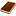國圖紙本論文推文當script無法執行時可按︰推文 網路書籤當script無法執行時可按︰網路書籤 推薦當script無法執行時可按︰推薦 評分當script無法執行時可按︰評分 引用網址當script無法執行時可按︰引用網址 轉寄當script無法執行時可按︰轉寄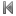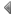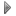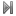top
 1 皇冠圖之小（有向）迴圈充填與覆蓋

 1 王麗雲（民91）。中文拼音政策的爭議與課程政治面向的反省，教育研究集刊，48（1）：95-131。 2 王麗雲（民91）。中文拼音政策的爭議與課程政治面向的反省，教育研究集刊，48（1）：95-131。 3 王麗雲（民91）。中文拼音政策的爭議與課程政治面向的反省，教育研究集刊，48（1）：95-131。 4 方永泉（民88）。文化研究與比較教育研究，比較教育，40，83-103頁。 5 方永泉（民88）。文化研究與比較教育研究，比較教育，40，83-103頁。 6 方永泉（民88）。文化研究與比較教育研究，比較教育，40，83-103頁。 7 孫紹誼（民84）。通俗文化、意識型態與話語霸權---伯明罕文化研究學派評述，當代，114，68-89頁。 8 孫紹誼（民84）。通俗文化、意識型態與話語霸權---伯明罕文化研究學派評述，當代，114，68-89頁。 9 孫紹誼（民84）。通俗文化、意識型態與話語霸權---伯明罕文化研究學派評述，當代，114，68-89頁。

 1 大三學生圖論概念學習之個案研究 2 互質圖的猜測 3 一維混沌系統 4 高維度網格型模型之花樣形成與置換矩陣 5 三環式網路Hyper-L型的研究 6 典型模型和單率模型間不阻塞性質蘊含關係之研究 7 利用折返理論研究含有長度較長迴圈的距離正則圖 8 量子運算上之多重目標搜尋演算法 9 Hermitian類型之Vogan圖 10 類神經網路的花式 11 NGARCH模型中選檡權之定價:應用於臺指選擇權 12 對多重標的物搜尋之Grover演算法的量子光學電路設計 13 離散型神經網路的動態行為 14 三級Clos網路在一對二傳播下之可重排性 15 灰階人臉辨識之研究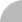簡易查詢 | 進階查詢 | 熱門排行 | 我的研究室